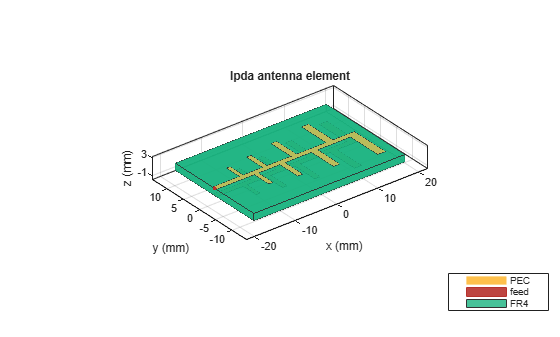Documentation

# lpda

Create printed log-periodic dipole array antenna

## Description

Use the `lpda` object to create a printed log-periodic dipole array antenna. The default antenna is centered at the origin and uses an FR4 substrate.## Creation

### Syntax

``lpdipole = lpda``
``lpdipole = lpda(Name,Value)``

### Description

example

````lpdipole = lpda` creates a printed log-periodic dipole array antenna using default property values.```
````lpdipole = lpda(Name,Value)` sets properties using one or more name-value pairs. For example, ```lpdipole = lpdipole('BoardLength',0.2)``` creates a printed log-periodic dipole array with a board length of 0.2 m. Enclose each property name in quotes.```

## Properties

expand all

Printed circuit board (PCB) length along X-axis, specified as a scalar in meters.

Example: `'BoardLength',0.2`

Example: `lpdipole.BoardLength = 0.2`

Data Types: `double`

PCB width along Y-axis, specified as a scalar in meters.

Example: `'BoardWidth',0.06`

Example: `lpdipole.BoardWidth = 0.06`

Data Types: `double`

PCB height along Z-axis, specified as a scalar in meters.

Example: `'Height',0.0018`

Example: `lpdipole.Height = 0.0018`

Data Types: `double`

Parallel strip line width, specified as a scalar in meters.

Example: `'StripLineWidth',0.0014`

Example: `lpdipole.StripLineWidth = 0.0014`

Data Types: `double`

Distance from edge feed point to smallest dipole, specified as a scalar in meters.

Example: `'FeedLength',0.0055`

Example: `lpdipole.FeedLength = 0.0055`

Data Types: `double`

Lengths of individual dipole arms, specified as a vector with each element unit in meters.

Example: ```'ArmLength',[0.0050 0.0055 0.0060 0.0066 0.0072 0.0079 0.0086 0.0095]```

Example: ```lpdipole.ArmLength = [0.0050 0.0055 0.0060 0.0066 0.0072 0.0079 0.0086 0.0095]```

Data Types: `double`

Widths of individual dipole arms, specified as a vector with each element unit in meters.

Example: ```'ArmWidth',[9.8000e-04 10.8000e-04 0.0021 0.0022 0.0023 0.0025 0.0027 0.0029]```

Example: ```lpdipole.ArmWidth = [9.8000e-04 10.8000e-04 0.0021 0.0022 0.0023 0.0025 0.0027 0.0029]```

Data Types: `double`

Spacing between individual dipole arms, specified as a vector with each element unit in meters.

Example: ```'ArmSpacing',[0.0037 0.0040 0.0043 0.0047 0.0051 0.0056 0.0061]```

Example: ```lpdipole.ArmSpacing = [0.0037 0.0040 0.0043 0.0047 0.0051 0.0056 0.0061]```

Data Types: `double`

Type of dielectric material used as a substrate, specified as an dielectric object. For more information, see `dielectric`. For more information on dielectric substrate meshing, see Meshing.

### Note

The substrate dimensions must be equal to the groundplane dimensions.

Example: ```d = dielectric('Teflon'); 'Substrate',d```

Example: ```d = dielectric('Teflon'); lpdipole.Substrate = d```

Lumped elements added to the antenna feed, specified as a lumped element object. You can add a load anywhere on the surface of the antenna. By default, the load is at the origin. For more information, see `lumpedElement`.

Example: `'Load',lumpedelement`, where `lumpedelement` is the object handle for the load created using `lumpedElement`.

Example: ```lpda.Load = lumpedElement('Impedance',75)```

Tilt angle of the antenna, specified as a scalar or vector with each element unit in degrees. For more information, see Rotate Antennas and Arrays.

Example: `'Tilt',90`

Example: `'Tilt',[90 90]`,`'TiltAxis',[0 1 0;0 1 1]` tilts the antenna at 90 degree about two axes, defined by vectors.

Data Types: `double`

Tilt axis of the antenna, specified as:

• Three-element vectors of Cartesian coordinates in meters. In this case, each vector starts at the origin and lies along the specified points on the X-, Y-, and Z-axes.

• Two points in space, each specified as three-element vectors of Cartesian coordinates. In this case, the antenna rotates around the line joining the two points in space.

• A string input describing simple rotations around one of the principal axes, 'X', 'Y', or 'Z'.

Example: `'TiltAxis',[0 1 0]`

Example: `'TiltAxis',[0 0 0;0 1 0]`

Example: `ant.TiltAxis = 'Z'`

## Object Functions

 `show` Display antenna or array structure; Display shape as filled patch `axialRatio` Axial ratio of antenna `beamwidth` Beamwidth of antenna `charge` Charge distribution on metal or dielectric antenna or array surface `current` Current distribution on metal or dielectric antenna or array surface `design` Design prototype antenna or arrays for resonance at specified frequency `EHfields` Electric and magnetic fields of antennas; Embedded electric and magnetic fields of antenna element in arrays `impedance` Input impedance of antenna; scan impedance of array `mesh` Mesh properties of metal or dielectric antenna or array structure `meshconfig` Change mesh mode of antenna structure `pattern` Radiation pattern and phase of antenna or array; Embedded pattern of antenna element in array `patternAzimuth` Azimuth pattern of antenna or array `patternElevation` Elevation pattern of antenna or array `returnLoss` Return loss of antenna; scan return loss of array `sparameters` S-parameter object `vswr` Voltage standing wave ratio of antenna

## Examples

collapse all

Create and view a printed log-periodic dipole array antenna.

`lpdipole = lpda`
```lpdipole = lpda with properties: BoardLength: 0.0366 BoardWidth: 0.0244 Height: 0.0016 StripLineWidth: 0.0012 FeedLength: 0.0065 ArmLength: [0.0040 0.0045 0.0050 0.0056 0.0062 0.0069 0.0076 0.0085] ArmWidth: [1x8 double] ArmSpacing: [0.0027 0.0030 0.0033 0.0037 0.0041 0.0046 0.0051] Substrate: [1x1 dielectric] Tilt: 0 TiltAxis: [1 0 0] Load: [1x1 lumpedElement] ```
`show(lpdipole)`